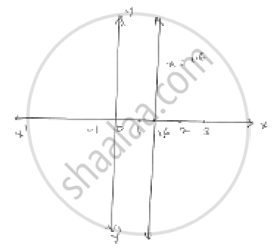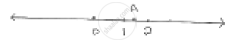# Give the Geometric Representation of the Following Equation (A) on the Number Line (B) on the Cartesian Plane : 3x – 5 = 0 - Mathematics

Give the geometric representation of the following equation
(a) on the number line     (b) on the Cartesian plane :   3x – 5 = 0

#### Solution3x - 5 = 0

3x = 5

 x = 5 / 3 = 1.6    (Approx )

Point A represents   1 1/2 (or)  5/3  Point A represents

On Cartesian plane, equation represents all points on  y -axis  for which  x = 16

Concept: Equations of Lines Parallel to the X-axis and Y-axis
Is there an error in this question or solution?

#### APPEARS IN

RD Sharma Mathematics for Class 9
Chapter 7 Linear Equations in Two Variables
Exercise 7.4 | Q 1.5

Share